# Deforming Surfaces According to Curve Wrapping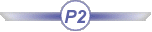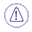This command is only available with the Generative Shape Optimizer product.This task shows how to deform surfaces basing the deformation on curve wrapping, that is matching each reference curve onto a target curve.
The deformation is then defined by the transformation of the reference curves into target curves.
The curves used for the deformation do not necessarily lie on the initial surface.

Several cases are presented here, from the simplest one to cases using various options. Note that whatever information is given in the first example also applies to the following examples.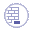Open the WrapCurve1.CATPart document.

### Basic Curve Wrapping Deformation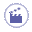1. Click WrapCurve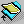.

 The Wrap Curve Definition dialog box is displayed.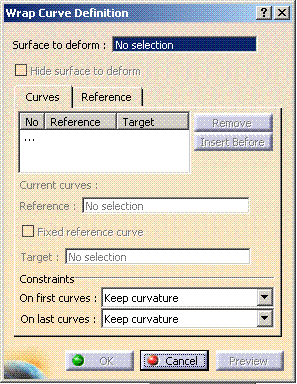2. Select the surface to be deformed.

3. Successively select the first reference curve and the first target curve.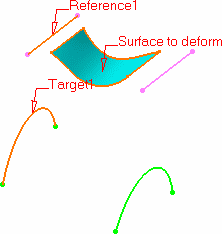4. Repeat this operation by selecting the second reference curve then the second target curve.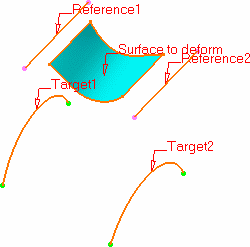As you select pairs of reference/target curves, the curves list in dialog box is updated accordingly.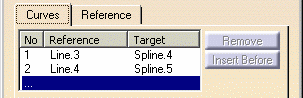5. Click OK to create the deformed surface.

 The element (identified as Wrap curve.xxx) is added to the specification tree.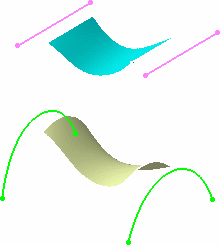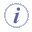You must always select successively a reference then a target curve to define a pair. You cannot select all reference curves, then all target curves for example. You need to select only one pair of curves (reference and target) to be able to define the deformation by clicking Preview. When several pairs of curves are selected, they must be ordered, not randomly selected Reference curves should not intersect each other, nor should the target curves should intersect each other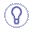Reference and target curves can be multi-cells. Joined, blended, or matched curves, for example, can be used as reference or target curves.

## Curve Wrapping Deformation with Fixed Reference

Some times you need to create a deformed surface in relation to another element, when you want to match two surfaces for example. The curve wrapping capability lets you fix an element that can be used by another one, thus allowing you to retain a connection between elements while deforming the initial surface.1. Click WrapCurve.

 The Wrap Curve Definition dialog box is displayed.
2. Successively select the surface to be deformed, and the first reference curve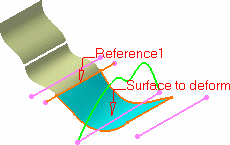3. Right-click in space to display the contextual menu, and choose the Fixed reference curve.

 The reference curve you selected previously now is fixed, i.e. you do not need a target curve, this curve being used to create the deformation. In the target area of the list, no element is displayed.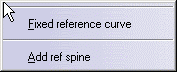4. Select another pair of reference and target curves and click Preview.

 A new surface is created based on the first reference curve and the second target curve.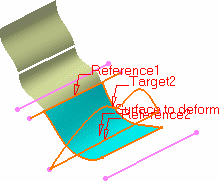5. Click OK to create the deformed surface.

 Because the first reference curve is an element used by the blended surface the connection between the two surfaces is retained.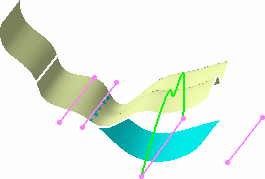## Editing a Deformed Surface

Using the deformed surface you just created, this section shows you how to modify it by:1. Double-click on the wrap curve surface you have just created.

 The dialog box is displayed containing the creation information.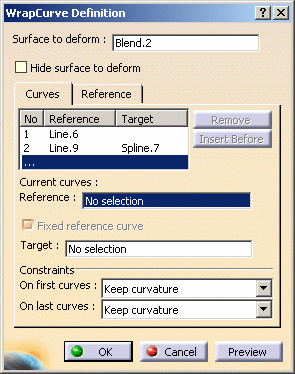2. Within the list, select the second line (Reference: Line.9, Target: Spline.7) and click Insert Before.

 The Reference field of the Current curves area gets active.
3. Select a new reference curve (Line.8) and a new target curve (Spline.6), and click Preview.

 The deformed surface now takes into account the new pair of curves.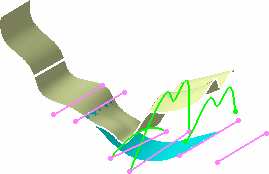To add a pair of curves as the last entry in the list, you need to select the ... line, and directly select the reference and target curves. In our example, we selected the ... line, then selected Line.7 and Spline.8 as reference and target curves respectively.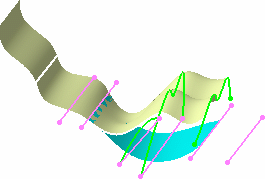Just like you fixed a reference curve at creation time, you can do it when editing a wrap curve surface.
4. Select the fourth line from the list in the dialog box, and check Fixed reference curve.

 The target curve is automatically removed from the Target column and field.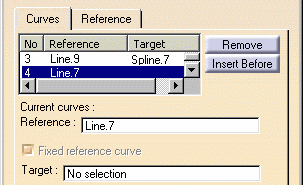5. Click Preview.

 The resulting surface looks like this: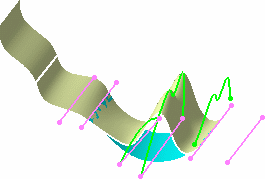6. Select the third line from the list in the dialog box, and click Remove, and click OK.

 The selected pair of curves no longer being used to compute the resulting surface, the latter looks like this: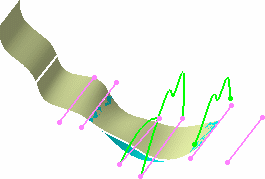You can define further constraints on the deformed surface by means of the Constraints fields. You can choose to retain the initial surface's curvature or tangency constraint on the first, and/or last; pair of curves. In case of a curvature or tangency continuity, the original continuity between the deformed area and the surface outside the deformation area will be at best kept but may be approximate in certain cases.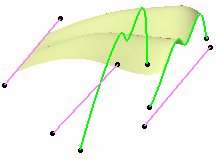Not keeping initial surface's constraint on last reference/target curves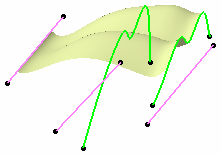Keeping initial surface's tangency constraint  on last reference/target curves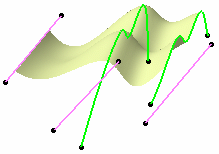Keeping initial surface's curvature continuity on last reference/target curvesWhen the spine or the first reference/target curve (default spine) is too short in relation to the corresponding surface's bounding box, the curve is extrapolated according to this bounding box. Then other reference/target curves are extrapolated as well, in relation to this extrapolated spine.

### How Is the Deformation Computed ?

The following diagrams will help you understand how the deformation is computed in relation to the entered data, i.e. reference/target curves and possible spine.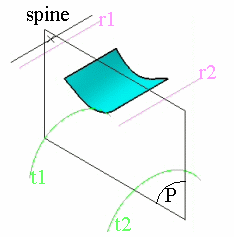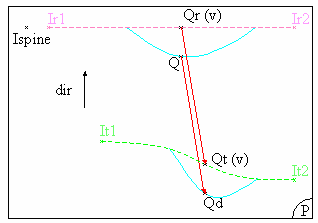3D view, where:
• r1, r2 are the reference curves
• t1, t2 are the target curves
• P is a plane normal to the spine
Planar view, where:
• Ir1: is the intersection between P and r1
• Ir2: is the intersection between P and r2
• It1: is the intersection between P and t1
• It2: is the intersection between P and t2

The deformation is computed in each plane P, normal to the spine. By default the spine is the first reference curve, but you can select a new spine using the Spine field in the Reference tab.

In each plane P, the system computes the intersection between the plane and each curve.
A curve (Cr) is created between the first intersection point (Ir1) and the last intersection point (Irn) on reference curves, passing through all the intersection points between these two.
Similarly, a curve (Ct) is created passing through all the intersections points between the first (It1) and the last intersection point (Itn) on target curves.

Then, for each point Q, resulting from the intersection of the surface to be deformed with the plane, Q is projected onto the curve Cr according to the projection direction (dir). This projection direction is the vectorial product of: vector(lspine, lr2) ^ vector normal to P.
The result of the projection of point Q is the point Qr, which parameter on Cr is v.
Similarly, a point Qt is created on the curve Ct, with the same v parameter as point Qr on curve Cr .

Then Qd, that is the transformation of point Q according to the wrap curve deformation, is obtained by adding: Q+vector(Qr,Qt)